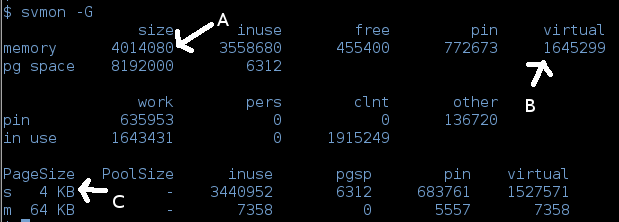##Because of AIX memory handling, it will used up all the available memory, allocating for program usage and file caching. Hence, you can't use the “inuse” figure as the program used memory.
A = total memory in block
B = total allocated virtual memory in block
C = page size / block size
Calculation:
Total memory = (4014080 * 4KB) / 1024KB = 15680.00MB
Total used memory = (1645299 * 4KB) / 1024KB = 6426.94MB
Total used memory in % = (1645299 / 4014080 ) * 100 = 40%

Total free memory = 15680.00MB – 6426.94MB = 9253.06MB